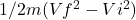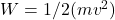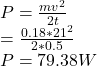## When a rattlesnake strikes, its head accelerates from rest to a speed of 21 m/s in 0.5 seconds. Assume for simplicity that the only moving p

Question

When a rattlesnake strikes, its head accelerates from rest to a speed of 21 m/s in 0.5 seconds. Assume for simplicity that the only moving part of the snake is its head of mass 180 g. How much (average) power does the rattlesnake need to accelerate its head that fast? Answer in units of W.

in progress 0
2 weeks 2021-08-30T19:22:14+00:00 2 Answers 0 views 0

Power = 79.38 W

Explanation:

Given Data:

Mass of the snake head = m = 180 g = 0.18 kg

Velocity of snake = v = 21 m/s

Time = t = 0.5 sec

Required:

Average Power = P = ?

Solution

Power = work done / time

Work done = W = change in Kinetic Energy =Since Vi = zero as snake was at rest at initial stage, thus

Work done =Substituting this value in power equation2. Explanation:

Below is an attachment containing the solution.